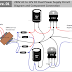Hey, in this article we are going to see the circuit diagram of a 12V DC dual power supply. The dual power supply is used in electronic testing, laboratories, amplifiers, etc. Here, we are going to see the circuit diagram that consists of very few components. Here, you will get up to 3A output current. The circuit is designed for an input power supply of 230V and output of +12V and -12V. You have to connect a load between the +12V and 0V terminal to get the +12V DC supply and between -12V and 0V terminal to get the -12V DC supply. In fact, you will get a 24V DC power output between +12V and -12V terminals. It has three output terminals - 1. +12V 2. 0V and 3. -12V.

## 12V DC Dual Power Supply Circuit Diagram

Here, you can see the circuit diagram of the 12V DC duel power supply with 230V AC input and the connection between each component.

## List of Components Used

• 1 Nos 230V AC/12-0-12 Step Down Transformer
• 4 Nos 1N4007 PN Junction Diode
• 2 Nos 25V, 220uF Electrolytic Capacitor
• 1 Nos 7812 IC
• 1 Nos 7912 IC

## Connection Procedure

1. Connect a 1N4007 Diode to any one 12V terminal of the transformer in forward bias.
2. Connect the output of that diode to the positive terminal of a 25V capacitor.
3. Connect the same terminal(positive terminal of the capacitor) to the Input terminal(1) of the IC 7812.
4. Connect the negative terminal of that capacitor and ground terminal(2) of that 7812IC to the 0V terminal of the transformer.
5. Brought out the output terminal(3) of the 7812 IC as the +12V DC output terminal.
6. Now, connect a 1N4007 Diode to another 12V terminal of the transformer in reverse bias.
7. Connect another terminal of that diode to the negative terminal of another 25V capacitor.
8. Connect the same terminal (negative terminal of the capacitor) to the input terminal(2) of the IC 7912.
9. Connect the positive terminal of the Capacitor and Ground terminal(1) of the IC 7912 to the 0V terminal of the transformer.
10. Brought out the output terminal (3) of the IC 7912 as -12V DC output.
11. Brought out the 0V terminal of the transformer as the output ground terminal.
12. Connect a 1N4007 diode to the 12V terminal of the transformer(where already a diode is connected in forward bias) in reverse bias and connect another terminal of that diode to the negative terminal of the second capacitor.
13. Connect a 1N4007 diode to the 12V terminal of the transformer(where already a diode is connected in reverse bias) in forward bias and connect another terminal of that diode to the positive terminal of the first capacitor.
14. The input terminal of the transformer is to be connected to the 230V AC power source while output is required.

## Working Principle

The transformer steps down the 230V AC voltage into a splitter 12V AC voltage. Here, we will get 12V AC voltage between 0V and any 12V terminal and will get 24V AC voltage between two 12V terminals.

After the 1N4007 diodes form a bridge rectifier circuit that converts the positive half cycle into +12V DC and the negative half cycle into -12V DC.

Now we got both +12V and -12V DC power supply but they are not filtered. So the two 25V electrolytic capacitors are used to filter the DC from the output of the rectifier. One capacitor filters the +12V supply while another filters the -12V supply.

Nw, we need to regulate that DC power supply to get a regulated stable output power. So here IC 7812 and IC 7912 are used to regulate the DC power supply. The IC7812 regulates the +12V supply while IC 7912 regulates the -12V supply.

230V AC to 12V DC Dual Power Supply Circuit DiagramReviewed by Author on April 29, 2023 Rating: 5